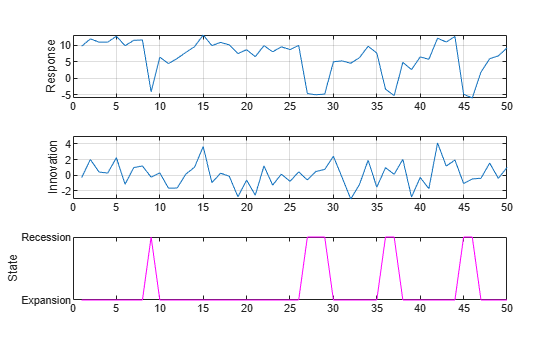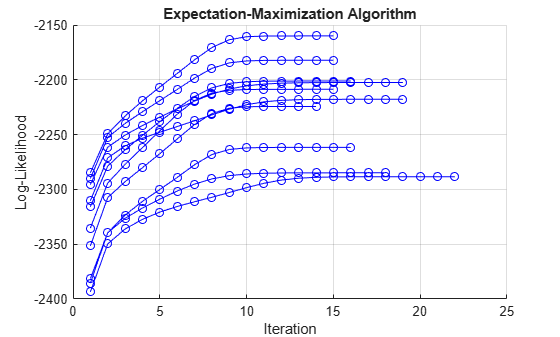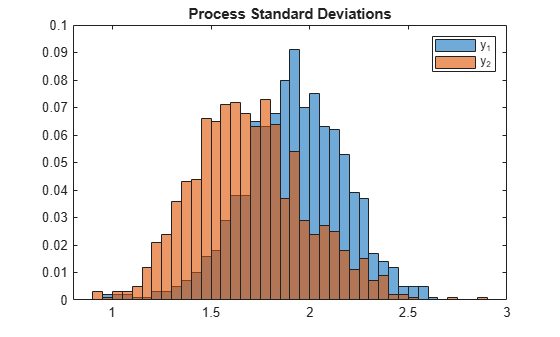# simulate

Simulate sample paths of Markov-switching dynamic regression model

## Syntax

``Y = simulate(Mdl,numObs)``
``Y = simulate(Mdl,numObs,Name,Value)``
``[Y,E,StatePaths] = simulate(___)``

## Description

example

``Y = simulate(Mdl,numObs)` returns a random `numObs`-period path of response series `Y` from simulating the fully specified Markov-switching dynamic regression model `Mdl`.`

example

````Y = simulate(Mdl,numObs,Name,Value)` uses additional options specified by one or more name-value arguments. For example, `'NumPaths',1000,'Y0',Y0` simulates `1000` sample paths and initializes the dynamic component of each submodel by using the presample response data `Y0`.```

example

``[Y,E,StatePaths] = simulate(___)` also returns the simulated innovation paths `E` and the simulated state paths `StatePaths`, using any of the input argument combinations in the previous syntaxes. `

## Examples

collapse all

Simulate a response path from a two-state Markov-switching dynamic regression model for a 1-D response process. This example uses arbitrary parameter values.

Create Fully Specified Model

Create a two-state discrete-time Markov chain model that describes the regime switching mechanism. Label the regimes.

```P = [0.9 0.1; 0.3 0.7]; mc = dtmc(P,'StateNames',["Expansion" "Recession"]);```

`mc` is a fully specified `dtmc` object.

For each regime, use arima to create an AR model that describes the response process within the regime. Store the submodels in a vector.

```mdl1 = arima('Constant',5,'AR',[0.3 0.2],... 'Variance',2); mdl2 = arima('Constant',-5,'AR',0.1,... 'Variance',1); mdl = [mdl1; mdl2];```

`mdl1` and `mdl2` are fully specified `arima` objects.

Create a Markov-switching dynamic regression model from the switching mechanism `mc` and the vector of submodels `mdl`.

`Mdl = msVAR(mc,mdl);`

`Mdl` is a fully specified `msVAR` object.

Simulate Response Path

Generate one random response path of length 50 from the model.

```rng(1); % For reproducibility y = simulate(Mdl,50);```

`y` is a 50-by-1 vector of one response path.

Plot the response path.

```figure plot(y) xlabel("Time") ylabel("Response")```Consider the model in Simulate Response Path.

Create the Markov-switching dynamic regression model.

```P = [0.9 0.1; 0.3 0.7]; mc = dtmc(P,'StateNames',["Expansion" "Recession"]); mdl1 = arima('Constant',5,'AR',[0.3 0.2],... 'Variance',2); mdl2 = arima('Constant',-5,'AR',0.1,... 'Variance',1); mdl = [mdl1; mdl2]; Mdl = msVAR(mc,mdl);```

Simulate 3 response, innovations, and state-index paths of 5 observations from the model.

```rng('default') % For reproducibility [Y,E,SP] = simulate(Mdl,5,'NumPaths',3)```
```Y = 5×3 -5.7605 10.9496 11.4633 -5.7002 8.5772 -3.1268 4.2446 10.7774 -5.6161 -3.1665 -2.2920 -5.2677 -3.8995 -4.7403 -6.3141 ```
```E = 5×3 -0.2050 0.9496 1.4633 -0.1241 -1.7076 0.7269 2.1068 1.0143 -0.3034 1.4090 1.6302 0.2939 1.4172 0.4889 -0.7873 ```
```SP = 5×3 2 1 1 2 1 2 1 1 2 2 2 2 2 2 2 ```

`Y`, `E`, and `SP` are 5-by-3 matrices. Columns represent separate, independent paths.

Simulate a single path of responses, innovations, and states into a simulation horizon of length 50. Then plot each path separately.

```[y,e,sp] = simulate(Mdl,50); figure subplot(3,1,1) plot(y) ylabel('Response') grid on subplot(3,1,2) plot(e) ylabel('Innovation') grid on subplot(3,1,3) plot(sp,'m') ylabel('State') yticks([1 2]) yticklabels(Mdl.StateNames)```Consider a two-state Markov-switching dynamic regression model of the postwar US real GDP growth rate. The model has the parameter estimates presented in .

Create a discrete-time Markov chain model that describes the regime switching mechanism. Label the regimes.

```P = [0.92 0.08; 0.26 0.74]; mc = dtmc(P,'StateNames',["Expansion" "Recession"]);```

Create separate AR(0) models (constant only) for the two regimes.

```sigma = 3.34; % Homoscedastic models across states mdl1 = arima('Constant',4.62,'Variance',sigma^2); mdl2 = arima('Constant',-0.48,'Variance',sigma^2); mdl = [mdl1 mdl2];```

Create the Markov-switching dynamic regression model that describes the behavior of the US GDP growth rate.

`Mdl = msVAR(mc,mdl);`

`Mdl` is a fully specified `msVAR` object.

Generate one random path of 100 responses, corresponding innovations, and states from the model.

```rng(1) % For reproducibility [y,e,sp] = simulate(Mdl,100);```

`y` is a 100-by-1 vector of GDP rates, and `e` is a 100-by-1 vector of corresponding innovations. `sp` is a 100-by-1 vector of state indices.

Consider the Markov-switching model in Simulate US GDP Rates and Economic States, but assume that the submodels are AR(1) instead. Consider fitting the model to observations in the period 1960:Q1–2004:Q2.

Create the model template for estimation. Specify AR(1) submodels.

```mc = dtmc(NaN(2),'StateNames',["Expansion" "Recession"]); ar1 = arima(1,0,0); Mdl = msVAR(mc,[ar1; ar1]);```

Because the submodels are AR(1), each requires one presample observation to initialize its dynamic component for estimation.

Create the model containing initial parameter values for the estimation procedure.

```mc0 = dtmc(0.5*ones(2),'StateNames',["Expansion" "Recession"]); submdl01 = arima('Constant',1,'Variance',1,'AR',0.001); submdl02 = arima('Constant',-1,'Variance',1,'AR',0.001); Mdl0 = msVAR(mc0,[submdl01; submdl02]);```

Load the data. Transform the entire set to an annualized rate series.

```load Data_GDP qrate = diff(Data)./Data(1:(end - 1)); arate = 100*((1 + qrate).^4 - 1);```

Identify the presample and estimation sample periods using the dates associated with the annualized rate series. Because the transformation applies the first difference, you must drop the first observation date from the original sample.

```dates = datetime(dates(2:end),'ConvertFrom','datenum',... 'Format','yyyy:QQQ','Locale','en_US'); estPrd = datetime(["1960:Q2" "2004:Q2"],'InputFormat','yyyy:QQQ',... 'Format','yyyy:QQQ','Locale','en_US'); idxEst = isbetween(dates,estPrd(1),estPrd(2)); idxPre = dates < estPrd(1);```

Fit the model to the estimation sample data. Specify the presample observation.

```y0 = arate(idxPre); EstMdl = estimate(Mdl,Mdl0,arate(idxEst),'Y0',y0);```

Simulate a response path from the fitted model over the estimation period. Specify the presample observation.

```rng(1) % For reproducibility numObs = sum(idxEst); aratesim = simulate(EstMdl,numObs,'Y0',y0);```

Plot the observations and simulated path of annualized rates, and identify periods of recession by using `recessionplot`.

```figure; plot(dates(idxEst),[arate(idxEst) aratesim]) recessionplot xlabel("Time") ylabel("Annualized GDP Rate") legend("Observed","Simulated");```Assess estimation accuracy using simulated data from a known data-generating process (DGP). This example uses arbitrary parameter values.

Create Model for DGP

Create a fully specified, two-state discrete-time Markov chain model for the switching mechanism.

```P = [0.7 0.3; 0.1 0.9]; mc = dtmc(P);```

For each state, create a fully specified AR(1) model for the response process.

```% Constants C1 = 5; C2 = -2; % Autoregression coefficients AR1 = 0.4; AR2 = 0.2; % Variances V1 = 4; V2 = 2; % AR Submodels dgp1 = arima('Constant',C1,'AR',AR1,'Variance',V1); dgp2 = arima('Constant',C2,'AR',AR2,'Variance',V2);```

Create a fully specified Markov-switching dynamic regression model for the DGP.

`DGP = msVAR(mc,[dgp1,dgp2]);`

Simulate Response Paths from DGP

Generate 10 random response paths of length 1000 from the DGP.

```rng(1); % For reproducibility N = 10; n = 1000; Data = simulate(DGP,n,'Numpaths',N);```

`Data` is a 1000-by-10 matrix of simulated responses.

Create Model for Estimation

Create a partially specified Markov-switching dynamic regression model that has the same structure as the data-generating process, but specify an unknown transition matrix and unknown submodel coefficients.

```PEst = NaN(2); mcEst = dtmc(PEst); mdl = arima(1,0,0); Mdl = msVAR(mcEst,[mdl; mdl]);```

Create Model Containing Initial Values

Create a fully specified Markov-switching dynamic regression model that has the same structure as `Mdl`, but set all estimable parameters to initial values.

```P0 = 0.5*ones(2); mc0 = dtmc(P0); mdl01 = arima('Constant',1,'AR',0.5,'Variance',2); mdl02 = arima('Constant',-1,'AR',0.5,'Variance',1); Mdl0 = msVAR(mc0,[mdl01,mdl02]);```

Estimate Models

Fit the model to each simulated path. For each path, plot the loglikelihood at each iteration of the EM algorithm.

```c1 = zeros(N,1); c2 = zeros(N,1); v1 = zeros(N,1); v2 = zeros(N,1); ar1 = zeros(N,1); ar2 = zeros(N,1); PStack = zeros(2,2,N); figure hold on for i = 1:N EstModel = estimate(Mdl,Mdl0,Data(:,i),'IterationPlot',true); c1(i) = EstModel.Submodels(1).Constant; c2(i) = EstModel.Submodels(2).Constant; v1(i) = EstModel.Submodels(1).Covariance; v2(i) = EstModel.Submodels(2).Covariance; ar1(i) = EstModel.Submodels(1).AR{1}; ar2(i) = EstModel.Submodels(2).AR{1}; PStack(:,:,i) = EstModel.Switch.P; end hold off```Assess Accuracy

Compute the Monte Carlo mean of each estimated parameter.

```c1Mean = mean(c1); c2Mean = mean(c2); v1Mean = mean(v1); v2Mean = mean(v2); ar1Mean = mean(ar1); ar2Mean = mean(ar2); PMean = mean(PStack,3);```

Compare population parameters to the corresponding Monte Carlo estimates.

```DGPvsEstimate = [... C1 c1Mean C2 c2Mean V1 v1Mean V2 v2Mean AR1 ar1Mean AR2 ar2Mean]```
```DGPvsEstimate = 6×2 5.0000 5.0260 -2.0000 -1.9615 4.0000 3.9710 2.0000 1.9903 0.4000 0.4061 0.2000 0.2017 ```
`P`
```P = 2×2 0.7000 0.3000 0.1000 0.9000 ```
`PEstimate = PMean`
```PEstimate = 2×2 0.7065 0.2935 0.1023 0.8977 ```

Generate random paths from a three-state Markov-switching dynamic regression model for a 2-D VARX response process. This example uses arbitrary parameter values for the DGP.

Create Fully Specified Model for DGP

Create a three-state discrete-time Markov chain model for the switching mechanism.

```P = [10 1 1; 1 10 1; 1 1 10]; mc = dtmc(P);```

`mc` is a fully specified `dtmc` object. `dtmc` normalizes the rows of `P` so that they sum to `1`.

For each regime, use `varm` to create a VAR model that describes the response process within the regime. Specify all parameter values.

```% Constants C1 = [1;-1]; C2 = [2;-2]; C3 = [3;-3]; % Autoregression coefficients AR1 = {}; AR2 = {[0.5 0.1; 0.5 0.5]}; AR3 = {[0.25 0; 0 0] [0 0; 0.25 0]}; % Regression coefficients Beta1 = [1;-1]; Beta2 = [2 2;-2 -2]; Beta3 = [3 3 3;-3 -3 -3]; % Innovations covariances Sigma1 = [1 -0.1; -0.1 1]; Sigma2 = [2 -0.2; -0.2 2]; Sigma3 = [3 -0.3; -0.3 3]; % VARX submodels mdl1 = varm('Constant',C1,'AR',AR1,'Beta',Beta1,'Covariance',Sigma1); mdl2 = varm('Constant',C2,'AR',AR2,'Beta',Beta2,'Covariance',Sigma2); mdl3 = varm('Constant',C3,'AR',AR3,'Beta',Beta3,'Covariance',Sigma3); mdl = [mdl1; mdl2; mdl3];```

`mdl` contains three fully specified `varm` model objects.

For the DGP, create a fully specified Markov-switching dynamic regression model from the switching mechanism `mc` and the submodels `mdl`.

`Mdl = msVAR(mc,mdl);`

`Mdl` is a fully specified `msVAR` model.

Simulate Data Ignoring Regression Component

If you do not supply exogenous data, `simulate` ignores the regression components in the submodels. Simulate 3 separate, independent paths of responses, innovations, and state indices of length 5 from the model.

```rng(1); % For reproducibility [Y,E,SP] = simulate(Mdl,5,'NumPaths',3)```
```Y = Y(:,:,1) = 5.2387 1.5297 4.4290 4.2738 1.1668 -1.2905 -0.9654 -0.2028 -0.2701 0.8993 Y(:,:,2) = 2.7737 -2.5383 -0.8651 -1.1046 -0.0511 0.3696 0.5826 -0.8926 2.4022 -0.6912 Y(:,:,3) = 3.5443 0.8768 4.9748 -0.7956 5.7213 0.8073 4.2473 0.5805 2.7972 -1.3340 ```
```E = E(:,:,1) = 1.2387 1.5297 -0.3434 2.8896 0.1668 -0.2905 -1.9654 0.7972 -1.2701 1.8993 E(:,:,2) = 1.7737 -1.5383 -1.8651 -0.1046 -1.0511 1.3696 -0.4174 0.1074 1.4022 0.3088 E(:,:,3) = -0.4557 0.8768 1.1150 -1.0061 1.3134 0.7176 -0.6941 -0.6838 1.7972 -0.3340 ```
```SP = 5×3 2 1 2 2 1 2 1 1 2 1 1 2 1 1 1 ```

`Y` and `E` are 5-by-2-by-3 arrays of simulated responses and innovations, respectively. Rows correspond to time points, columns correspond to variables in the system, and pages correspond to paths. `SP` is a 5-by-3 matrix whose columns correspond to paths.

Simulate a single path of responses, innovations, and states into a simulation horizon of length 50. Then plot each path separately.

```rng0 = rng; % Store settings to reproduce state sequence. [Y,E,SP] = simulate(Mdl,50); figure subplot(3,1,1) plot(Y) ylabel("Response") grid on legend(["y_1" "y_2"]) subplot(3,1,2) plot(E) ylabel("Innovation") grid on legend(["e_1" "e_2"]) subplot(3,1,3) plot(SP,'m') ylabel("State") yticks([1 2 3])```Simulate Data Including Regression Component

Simulate exogenous data for the three regressors by generating 50 random observations from the 3-D standard Gaussian distribution.

`X = randn(50,3);`

Generate one random response, innovation, and state path of length 50. Specify the simulated exogenous data for the submodel regression components. Plot the results.

```rng(rng0); % Reproduce state sequence in previous simulation. [Y,E,SP] = simulate(Mdl,50,'X',X); figure subplot(3,1,1) plot(Y) ylabel("Response") grid on legend(["y_1" "y_2"]) subplot(3,1,2) plot(E) ylabel("Innovation") grid on legend(["e_1" "e_2"]) subplot(3,1,3) plot(SP,'m') ylabel("State") yticks([1 2 3])```Consider the model in Simulate Paths from Model with VARX Submodels.

Create Fully Specified Model

Create the Markov-switching model excluding the regression component.

```P = [10 1 1; 1 10 1; 1 1 10]; mc = dtmc(P); C1 = [1;-1]; C2 = [2;-2]; C3 = [3;-3]; AR1 = {}; AR2 = {[0.5 0.1; 0.5 0.5]}; AR3 = {[0.25 0; 0 0] [0 0; 0.25 0]}; Sigma1 = [1 -0.1; -0.1 1]; Sigma2 = [2 -0.2; -0.2 2]; Sigma3 = [3 -0.3; -0.3 3]; mdl1 = varm('Constant',C1,'AR',AR1,'Covariance',Sigma1); mdl2 = varm('Constant',C2,'AR',AR2,'Covariance',Sigma2); mdl3 = varm('Constant',C3,'AR',AR3,'Covariance',Sigma3); mdl = [mdl1; mdl2; mdl3]; Mdl = msVAR(mc,mdl);```

Simulate Multiple Paths

Generate 1000 random paths of responses for 50 time steps. Start all simulations at the first state.

```rng(10); % For reproducibility S0 = [1 0 0]; Y = simulate(Mdl,50,'S0',S0,'NumPaths',1000);```

`Y` is a 50-by-2-by-1000 array of simulated response paths.

Compute Monte Carlo Distribution

For each variable and path, compute the process mean.

`mus = mean(Y,1);`

For each variable, plot the Monte Carlo distribution of the process mean.

```figure h1 = histogram(mus(1,1,:),'Normalization',"probability",... 'BinWidth',0.1); hold on h2 = histogram(mus(1,2,:),'Normalization',"probability",... 'BinWidth',0.1); legend(["y_1" "y_2"]) title('Process Means') hold off```For each variable and path, compute the process standard deviation.

`sigmas = std(Y,0,1);`

For each variable, plot the Monte Carlo distribution of the process standard deviation.

```figure h1 = histogram(sigmas(1,1,:),'Normalization',"probability",... 'BinWidth',0.05); hold on h2 = histogram(sigmas(1,2,:),'Normalization',"probability",... 'BinWidth',0.05); legend(["y_1" "y_2"]) title('Process Standard Deviations') hold off```## Input Arguments

collapse all

Fully specified Markov-switching dynamic regression model, specified as an `msVAR` model object returned by `msVAR` or `estimate`. Properties of a fully specified model object do not contain `NaN` values.

Number of observations to generate for each sample path, specified as a positive integer.

Data Types: `double`

### Name-Value Arguments

Specify optional comma-separated pairs of `Name,Value` arguments. `Name` is the argument name and `Value` is the corresponding value. `Name` must appear inside quotes. You can specify several name and value pair arguments in any order as `Name1,Value1,...,NameN,ValueN`.

Example: `'NumPaths',1000,'Y0',Y0` simulates `1000` sample paths and initializes the dynamic component of each submodel by using the presample response data `Y0`.

Number of sample paths to generate, specified as the comma-separated pair consisting of `'NumPaths'` and a positive integer.

Example: `'NumPaths',1000`

Data Types: `double`

Presample response data, specified as the comma-separated pair consisting of `'Y0'` and a numeric matrix or array.

To use the same presample data for each `numPaths` path, specify a `numPreSampleObs`-by-`numSeries` matrix, where `numPaths` is the value of `NumPaths`, `numPreSampleObs` is the number of presample observations, and `numSeries` is the number of response variables.

To use different presample data for each path:

• For univariate ARX submodels, specify a `numPreSampleObs`-by-`numPaths` matrix.

• For multivariate VARX submodels, specify a `numPreSampleObs`-by-`numSeries`-by-`numPaths` array.

The number of presample observations `numPreSampleObs` must be sufficient to initialize the AR terms of all submodels. If `numPreSampleObs` exceeds the AR order of any state, `simulate` uses the latest observations.

Each time `simulate` switches states, it updates `Y0` using the latest simulated observations.

By default, `simulate` determines `Y0` by the submodel of the initial state (see `S0`):

• If the initial submodel is a stationary AR process without regression components, `simulate` sets presample observations to the unconditional mean.

• Otherwise, `simulate` sets presample observations to zero.

Data Types: `double`

Initial state probabilities, specified as the comma-separated pair consisting of `'S0'` and a nonnegative numeric vector of length `numStates`.

`simulate` normalizes `S0` to produce a distribution.

`simulate` selects the initial state of each path from `S0` at random. To start from a specific initial state, specify a distribution with a probability mass of `1` in that state.

By default, `simulate` sets `S0` to a steady-state distribution computed by `asymptotics`.

Example: `'S0',[0.2 0.2 0.6]`

Example: `'S0',[0 1]` specifies state 2 as the initial state.

Data Types: `double`

Predictor data used to evaluate regression components in all submodels of `Mdl`, specified as the comma-separated pair consisting of `'X'` and a numeric matrix or a cell vector of numeric matrices.

To use a subset of the same predictors in each state, specify `X` as a matrix with `numPreds` columns and at least `numObs` rows. Columns correspond to distinct predictor variables. Submodels use initial columns of the associated matrix, in order, up to the number of submodel predictors. The number of columns in the `Beta` property of `Mdl.SubModels(j)` determines the number of exogenous variables in the regression component of submodel `j`. If the number of rows exceeds `numObs`, then `simulate` uses the latest observations.

To use different predictors in each state, specify a cell vector of such matrices with length `numStates`.

By default, `simulate` ignores regression components in `Mdl`.

Data Types: `double`

## Output Arguments

collapse all

Simulated response paths, returned as a numeric matrix or array. `Y` represents the continuation of the presample responses in `Y0`.

For univariate ARX submodels, `Y` is a `numObs`-by-`numPaths` matrix. For multivariate VARX submodels, `Y` is a `numObs`-by-`numSeries`-by-`numPaths` array.

Simulated innovation paths, returned as a numeric matrix or array.

For univariate ARX submodels, `E` is a `numObs`-by-`numPaths` matrix. For multivariate VARX submodels, `E` is a `numObs`-by-`numSeries`-by-`numPaths` array.

Simulated state paths, returned as a `numObs`-by-`numPaths` numeric matrix.

 Chauvet, M., and J. D. Hamilton. "Dating Business Cycle Turning Points." In Nonlinear Analysis of Business Cycles (Contributions to Economic Analysis, Volume 276). (C. Milas, P. Rothman, and D. van Dijk, eds.). Amsterdam: Emerald Group Publishing Limited, 2006.

 Hamilton, J. D. "Analysis of Time Series Subject to Changes in Regime." Journal of Econometrics. Vol. 45, 1990, pp. 39–70.

 Hamilton, James D. Time Series Analysis. Princeton, NJ: Princeton University Press, 1994.